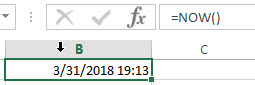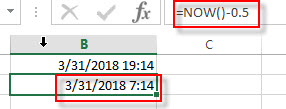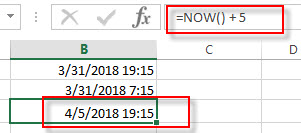# Excel NOW Function

This post will guide you how to use Excel NOW function with syntax and examples in Microsoft Excel.

### Description

The Excel NOW function returns the serial number of the current date and time. And it will be updated each time when your worksheet is changed or opened. This function do not need to add any arguments.

Before this function is entered into the Cell formula box, you need to change the cell format as date and time format if the cell format was General.

The Now function can be used to display the current date and time on your worksheet or use to calculate a value base on the current date and time.

The NOW function is a build-in function in Microsoft Excel and it is categorized as a DATE and TIME Function.

The NOW function is available in Excel 2016, Excel 2013, Excel 2010, Excel 2007, Excel 2003, Excel XP, Excel 2000, Excel 2011 for Mac.

### Syntax

The syntax of the NOW function is as below:

`=NOW ()`

Note:

• A serial date is how the Microsoft Excel stores dates and it represents the number of days since 1900-01-01, so the January 1, 1900 date is serial number 1 by default.
• Numbers to the right of the decimal point in the serial number represent the time; numbers to the left represent the date. For example, the serial number 0.5 represents the time 12:00 noon. And the serial number 0.25 represents the time 6:00 AM.
•  If the NOW function does not update cell values when you expect it to, you might need to change settings that control when the workbook or worksheet recalculates. These settings can be changed in Control Panel for the Excel desktop application.

### Excel NOW Function Examples

The below examples will show you how to use Excel NOW Function to return the current date and time.

1# get the current date and time, enter the following formula in Cell B1.

`=NOW()`2# get the date and time 12 hours ago, you can use the following formula:

`=NOW()-0.5`3# get the date and time 5 days in the future, just use the following formula:

`=NOW() + 5`### Related Functions

• Excel HOUR Function
The Excel HOUR function returns the hour of a time value. Or returns an integer value that represent the hour component of a given time. And the return value is between 0-23.The syntax of the HOUR function is as below:=HOUR (serial_number)…
• Excel SECOND Function
The Excel SECOND function returns the seconds of a time value. Or returns an integer value that represent the second component of a given excel time. And the return value is between 0-59.The syntax of the SECOND function is as below:=SECOND (serial_number)…
• Excel MINUTE Function
The Excel MINUTE function returns the minutes of a time value. And the minutes is an integer number from 0 to 59. The syntax of the MINUTE function is as below:=MINUTE (serial_number)…
• Excel DAY function
The Excel DAY function returns a day of a date (from 1 to 31). The DAY function is a build-in function in Microsoft Excel and it is categorized as a DATE and TIME Function.The syntax of the DAY function is as below:= DAY (date_value)
• Excel YEAR function
The Excel YEAR function returns a four-digit year from a given date value, the year is returned as an integer ranging from 1900 to 9999. The syntax of the YEAR function is as below:=YEAR (serial_number)…
• Excel MONTH function
The Excel MONTH function returns the month of a date represented by a serial number. And the month is an integer number from 1 to 12. The syntax of the MONTH function is as below:=MONTH (serial_number)…